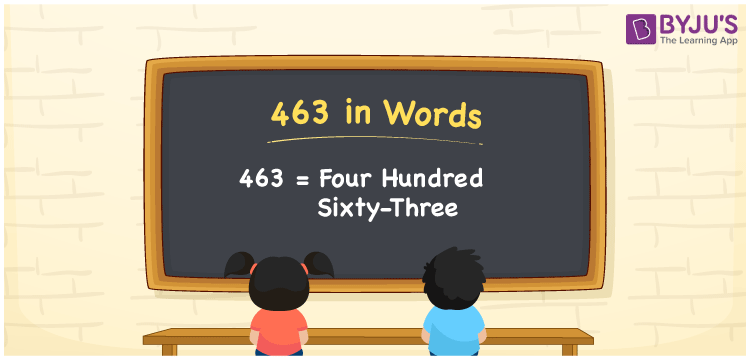# 463 in Words

463 in words is written as Four hundred sixty-three. In both the International System of Numerals and the Indian System of Numerals, 463 is written as Four hundred sixty-three. The number 463 is a Cardinal Number as it represents some quantity. For example, the “cost of the t-shirt is 463 rupees”.

 463 in Words Four hundred sixty-three Four hundred sixty-three in Number 463

## 463 in English Words

We write 463 in words using the letters of the English alphabet. Therefore, we read 463 in English as “Four hundred sixty-three”.## How to Write 463 in Words?

To write 463 in words, we shall use the place value chart. In the place value chart, write 4 in the hundreds, 6 in the tens, and 3 in the ones, respectively. Now let us make a place value chart to write the number 463 in words.

 Hundreds Tens Ones 4 6 3

Thus, we can write the expanded form as

4 × Hundred + 6 × Ten + 3 × One

= 4 × 100 + 6 × 10 + 3 × 1

= 400 + 60 + 3

= 463

= Four hundred sixty-three.

463 is a natural number, the successor of 462 and the predecessor of 464.

463 in words – Four hundred sixty-three

• Is 463 an odd number? – Yes
• Is 463 an even number? – No
• Is 463 a perfect square number? – No
• Is 463 a perfect cube number? – No
• Is 463 a prime number? – Yes
• Is 463 a composite number? – No

## Frequently Asked Questions on 463 in Words

Q1

### How to write 463 in words?

463 in words is written as Four hundred sixty-three.
Q2

### How to write 463 in the International and Indian System of Numerals?

In both, the system of numerals, 463 in words, is written as Four hundred sixty-three.
Q3

### What is the successor of 463?

The successor of 463 is 462.